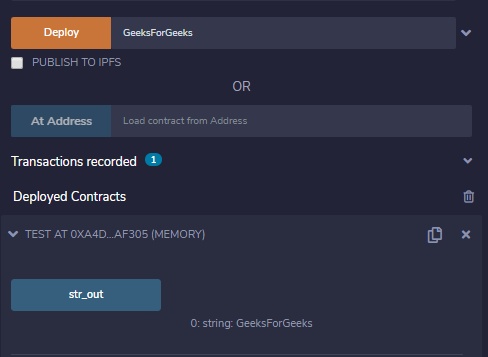# Solidity – Basics of Contracts

• Difficulty Level : Easy
• Last Updated : 11 May, 2022

Solidity Contracts are like a class in any other object-oriented programming language. They firmly contain data as state variables and functions which can modify these variables. When a function is called on a different instance (contract), the EVM function call happens and the context is switched in such a way that the state variables are inaccessible. A contract or its function needs to be called for anything to happen. Some basic properties of contracts are as follows :

• Constructor: Special method created using the constructor keyword, which is invoked only once when the contract is created.
• State Variables: These are the variables that are used to store the state of the contract.
• Functions: Functions are used to manipulate the state of the contracts by modifying the state variables.

### Creating a Contract

Creating contracts programmatically is generally done by using JavaScript API web3.js, which has a built-in function web3.eth.Contract to create the contracts. When a contract is created its constructor is executed, a constructor is an optional special method defined by using the constructor keyword which executes one per contract. Once the constructor is called the final code of the contract is added to the blockchain.

Syntax:

```contract <contract_name>{

constructor() <visibility>{
.......
}
// rest code
}
```

Example: In the below example, the contract Test is created to demonstrate how to create a contract in Solidity.

## Solidity

 `// Solidity program to demonstrate ``// how to create a contract``pragma solidity ^0.4.23;`` ` `// Creating a contract``contract` `Test {``   ` `  ``// Declaring variable``  ``string str;``  ` `  ``// Defining a constructor``  ``constructor(string str_in){``      ``str = str_in;``  ``}`` ` `  ``// Defining a function to ``  ``// return value of variable 'str'``  ``function str_out(``  ``) ``public` `view returns(string memory){``      ``return` `str;``  ``}``}`

Output :### Visibility Modifiers

Solidity provides four types of visibilities for functions and state variables. Functions have to specified by any of the four visibilities but for state variables external is not allowed.

1. External: External functions are can be called by other contracts via transactions. An external function cannot be called internally. For calling an external function within the contract this.function_name() method is used. Sometimes external functions are more efficient when they have large arrays of data.
2. Public: Public functions or variables can be called both externally or internally via messages. For public static variables, a getter method is created automatically in solidity.
3. Internal: These functions or variables can be accessed only internally i.e. within the contract or the derived contracts.
4. Private: These functions or variables can only be visible for the contracts in which they are defined. They are not accessible to derived contracts also.

Example: In the below example, the contract contract_example is created to demonstrate different visibility modifiers discussed above.

## Solidity

 `// Solidity program to demonstrate ``// visibility modifiers``pragma solidity ^0.5.0;`` ` `// Creating a contract``contract` `contract_example {`` ` `   ``// Declaring private ``   ``// state variable``   ``uint ``private` `num1;``    ` `   ``// Declaring public ``   ``// state variable``   ``uint ``public` `num2;`` ` `  ``// Declaring Internal ``  ``// state variable``   ``string internal str;`` ` `   ``// Defining a constructor``   ``constructor() ``public` `{``      ``num2 = 10;``   ``}`` ` `   ``// Defining a private function``   ``function increment(``     ``uint data1) ``private` `pure returns(``     ``uint) { ``return` `data1 + 1; }``    ` `   ``// Defining public functions``   ``function updateValue(``     ``uint data1) ``public` `{ num1 = data1; }``   ``function getValue(``   ``) ``public` `view returns(``     ``uint) { ``return` `num1; }`` ` `   ``// Declaring public functions``   ``function setStr(``     ``string memory _str) ``public``;``   ``function getStr(``   ``) ``public` `returns (string memory);`` ``}`` ` `   ``// Child contract inheriting ``   ``// from the parent contract``   ``// 'contract_example'``   ``contract` `derived_contract is contract_example{`` ` `   ``// Defining public function of ``   ``// parent contract``   ``function setStr(``     ``string memory _str) ``public``{``   ``str = _str;``   ``}`` ` `  ``// Defining public function ``  ``// of parent contract   ``  ``function getStr(``  ``) ``public` `returns (``    ``string memory){ ``return` `str; }``}`` ` `//External Contract``contract` `D {`` ` `   ``// Defining a public function to create ``   ``// an object of child contract access the``   ``// functions from child and parent contract``   ``function readData(``   ``) ``public` `payable returns(``     ``string memory, uint) {``      ``contract_example c ``        ``= ``new` `derived_contract();``     ``c.setStr(``"GeeksForGeeks"``);``      ``c.updateValue(16);         ``      ``return` `(c.getStr(), c.getValue());``   ``}``}`

Output :My Personal Notes arrow_drop_up